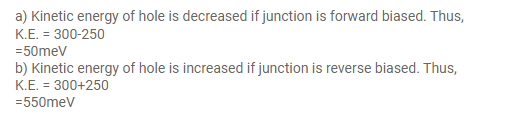# In a p-n junction, a potential barrier of 250meV

Question:

In a p-n junction, a potential barrier of $250 \mathrm{meV}$ exists across the junction. A hole with a kinetic energy of $300 \mathrm{meV}$ approaches the junction. Find the kinetic energy of the hole when it crosses the junction if the hole approached the junction

(a) from the p-side and

(b) from the n-side.

Solution: